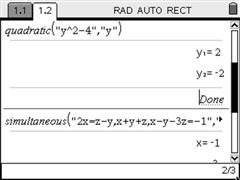PT EN

## Systems of Equations and Equations of 2nd DegreeTo install this library simply copy it to the Mylib folder and then select Refresh Libraries. Then you can access the new functions from the Catalog.

It has two functions: quadratic, to solve equations of the second degree and simultaneous, to solve systems of linear equations.

The simultaneous function solves systems of equations using a more straightforward notation: equations are entered in quotation marks, separated by commas, and the variables are entered in the same way. If an equation is entered without the = character, it's assumed that the right hand side is zero.

The quadratic function returns the real or complex solutions of any 2nd degree equation and detects double roots. If no real solutions exist and the complex numbers are set to Real on the document's settings, an error message is displayed. The notation is the same as for the simultaneous function: the equation is entered inside quotes followed by the variable.

This library isn't needed on TI-Nspire CAS. On TI-Nspire CAS you can use the solve function to solve equations or systems of equations.